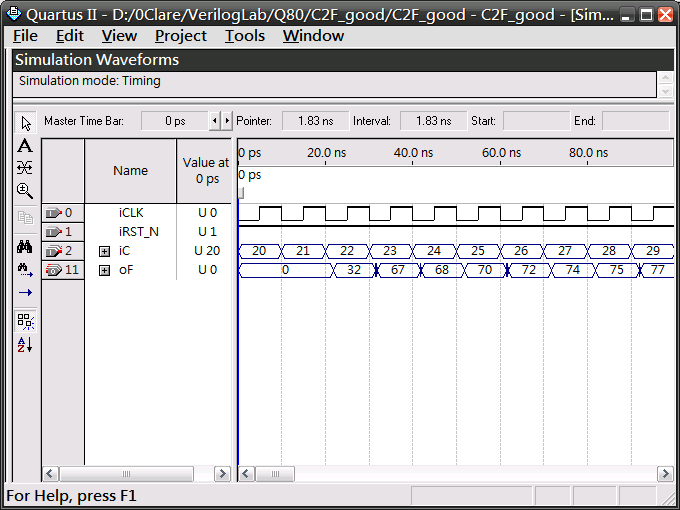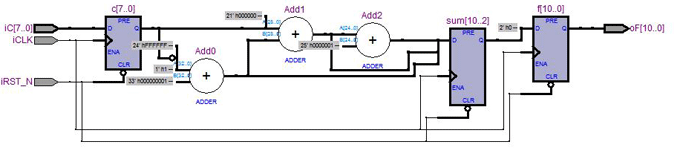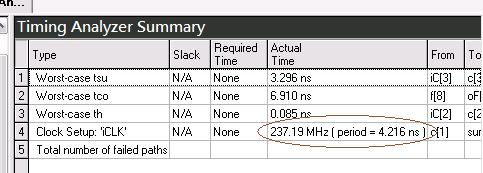## (原創) 如何計算浮點數? (SOC) (Verilog)

Abstract

Introduction

R = 1.164(Y-16) + 1.596(Cr-128)
G
= 1.164(Y-16) - 0.391(Cb-128) - 0.813(Cr-128)
B
= 1.164(Y-16) + 2.018(Cb-128)

F = C * 1.8 + 32

C為攝氏溫度，F為華氏溫度，我們來看看如何用Verilog實現。

2   input         iCLK,
3   input         iRST_N,
4   input  [7:0]  iC,
5   output [10:0] oF
6 );
7
8 reg [7:0]  c;
9 reg [10:0] f;
10
11 assign oF = f;
12
13 always@(posedge iCLK, negedge iRST_N) begin
14   if (!iRST_N) begin
15     c <= 0;
16     f <= 0;
17   end
18   else begin
19     f <= c * 1.8 + 32;
20   end
21 end
22
23 endmodule

Quartus II編譯後，出現以下錯誤訊息：

Error (10125): Verilog HDL error at C2F_bad.v(19): must use only constant operands for operator "*"

C2F_good.v / Verilog

1 /*
2 (C) OOMusou 2008 http://oomusou.cnblogs.com
3
4 Filename    : C2F_good.v
5 Compiler    : Quartus II 8.0
6 Description : Demo how to calculate float;
7 Release     : 10/11/2008 1.0
8 */
9
10 module C2F_good (
11   input         iCLK,
12   input         iRST_N,
13   input  [7:0]  iC,
14   output [10:0] oF
15 );
16
17 reg [7:0]  c;
18 reg [10:0] f;
19 reg [10:0] sum;
20
21 assign oF = f;
22
23 always@(posedge iCLK, negedge iRST_N) begin
24   if (!iRST_N) begin
25     c   <= 0;
26     f   <= 0;
27     sum <= 0;
28   end
29   else begin
30     c   <= iC;
31     sum <= c * 7 + 128;
32     f   <= sum >> 2;
33   end
34 end
35
36 endmoduleFmax為237.19MHz31行

sum <= c * 7 + 128;
f
<= sum >> 2;

F = C * 1.8 + 32

F << 2 = C * 7 + 128

32行

f   <= sum >> 2;

C2F_good.7z

Conclusion

posted on 2008-10-11 22:22 真 OO无双 阅读(...) 评论(...) 编辑 收藏